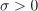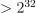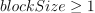# PostAnalyticalSimulation¶

class PostAnalyticalSimulation(*args)

Post analytical simulation.

Notes

This class inherits from EventSimulation.

The principle is to perform a simulation study to evaluate the threshold exceedance probability according to an importance density centered around the design point, in the standard space. The importance distribution is the standard distribution of the standard space.

A PostAnalyticalSimulation object can be created only through its derivative classes : PostAnalyticalImportanceSampling or PostAnalyticalControlledImportanceSampling.

Methods

 Draw the probability convergence at a given level. Accessor to the analytical result. Accessor to the block size. Accessor to the object's name. Accessor to the convergence strategy. Accessor to the event. Accessor to the object's id. Accessor to the maximum coefficient of variation. Accessor to the maximum sample size. Accessor to the maximum standard deviation. Accessor to the object's name. Accessor to the results. Accessor to the object's shadowed id. Accessor to verbosity. Accessor to the object's visibility state. Test if the object is named. Test if the object has a distinguishable name. Launch simulation. setBlockSize(blockSize) Accessor to the block size. setConvergenceStrategy(convergenceStrategy) Accessor to the convergence strategy. Accessor to the maximum coefficient of variation. setMaximumOuterSampling(maximumOuterSampling) Accessor to the maximum sample size. Accessor to the maximum standard deviation. setName(name) Accessor to the object's name. Set up a progress callback. Accessor to the object's shadowed id. setStopCallback(*args) Set up a stop callback. setVerbose(verbose) Accessor to verbosity. setVisibility(visible) Accessor to the object's visibility state.
 getControlProbability
__init__(*args)
drawProbabilityConvergence(*args)

Draw the probability convergence at a given level.

Parameters:
levelfloat, optional

The probability convergence is drawn at this given confidence length level. By default level is 0.95.

Returns:
graph

probability convergence graph

getAnalyticalResult()

Accessor to the analytical result.

Returns:
resultAnalyticalResult

Result of the analytical study which has been performed just before the simulation study centered around the importance factor.

getBlockSize()

Accessor to the block size.

Returns:
blockSizeint

Number of terms in the probability simulation estimator grouped together. It is set by default to 1.

getClassName()

Accessor to the object’s name.

Returns:
class_namestr

The object class name (object.__class__.__name__).

getConvergenceStrategy()

Accessor to the convergence strategy.

Returns:
storage_strategyHistoryStrategy

Storage strategy used to store the values of the probability estimator and its variance during the simulation algorithm.

getEvent()

Accessor to the event.

Returns:
eventRandomVector

Event we want to evaluate the probability.

getId()

Accessor to the object’s id.

Returns:
idint

Internal unique identifier.

getMaximumCoefficientOfVariation()

Accessor to the maximum coefficient of variation.

Returns:
coefficientfloat

Maximum coefficient of variation of the simulated sample.

getMaximumOuterSampling()

Accessor to the maximum sample size.

Returns:
outerSamplingint

Maximum number of groups of terms in the probability simulation estimator.

getMaximumStandardDeviation()

Accessor to the maximum standard deviation.

Returns:
sigmafloat,Maximum standard deviation of the estimator.

getName()

Accessor to the object’s name.

Returns:
namestr

The name of the object.

getResult()

Accessor to the results.

Returns:
resultsSimulationResult

Structure containing all the results obtained after simulation and created by the method run().

Accessor to the object’s shadowed id.

Returns:
idint

Internal unique identifier.

getVerbose()

Accessor to verbosity.

Returns:
verbosity_enabledbool

If True, the computation is verbose. By default it is verbose.

getVisibility()

Accessor to the object’s visibility state.

Returns:
visiblebool

Visibility flag.

hasName()

Test if the object is named.

Returns:
hasNamebool

True if the name is not empty.

hasVisibleName()

Test if the object has a distinguishable name.

Returns:
hasVisibleNamebool

True if the name is not empty and not the default one.

run()

Launch simulation.

Notes

It launches the simulation and creates a SimulationResult, structure containing all the results obtained after simulation. It computes the probability of occurrence of the given event by computing the empirical mean of a sample of size at most outerSampling * blockSize, this sample being built by blocks of size blockSize. It allows one to use efficiently the distribution of the computation as well as it allows one to deal with a sample sizeby a combination of blockSize and outerSampling.

setBlockSize(blockSize)

Accessor to the block size.

Parameters:
blockSizeint,Number of terms in the probability simulation estimator grouped together. It is set by default to 1.

Notes

For Monte Carlo, LHS and Importance Sampling methods, this allows one to save space while allowing multithreading, when available we recommend to use the number of available CPUs; for the Directional Sampling, we recommend to set it to 1.

setConvergenceStrategy(convergenceStrategy)

Accessor to the convergence strategy.

Parameters:
storage_strategyHistoryStrategy

Storage strategy used to store the values of the probability estimator and its variance during the simulation algorithm.

setMaximumCoefficientOfVariation(maximumCoefficientOfVariation)

Accessor to the maximum coefficient of variation.

Parameters:
coefficientfloat

Maximum coefficient of variation of the simulated sample.

setMaximumOuterSampling(maximumOuterSampling)

Accessor to the maximum sample size.

Parameters:
outerSamplingint

Maximum number of groups of terms in the probability simulation estimator.

setMaximumStandardDeviation(maximumStandardDeviation)

Accessor to the maximum standard deviation.

Parameters:
sigmafloat,Maximum standard deviation of the estimator.

setName(name)

Accessor to the object’s name.

Parameters:
namestr

The name of the object.

setProgressCallback(*args)

Set up a progress callback.

Can be used to programmatically report the progress of a simulation.

Parameters:
callbackcallable

Takes a float as argument as percentage of progress.

Examples

>>> import sys
>>> import openturns as ot
>>> experiment = ot.MonteCarloExperiment()
>>> X = ot.RandomVector(ot.Normal())
>>> Y = ot.CompositeRandomVector(ot.SymbolicFunction(['X'], ['1.1*X']), X)
>>> event = ot.ThresholdEvent(Y, ot.Less(), -2.0)
>>> algo = ot.ProbabilitySimulationAlgorithm(event, experiment)
>>> algo.setMaximumOuterSampling(100)
>>> algo.setMaximumCoefficientOfVariation(-1.0)
>>> def report_progress(progress):
...     sys.stderr.write('-- progress=' + str(progress) + '%\n')
>>> algo.setProgressCallback(report_progress)
>>> algo.run()


Accessor to the object’s shadowed id.

Parameters:
idint

Internal unique identifier.

setStopCallback(*args)

Set up a stop callback.

Can be used to programmatically stop a simulation.

Parameters:
callbackcallable

Returns an int deciding whether to stop or continue.

Examples

Stop a Monte Carlo simulation algorithm using a time limit

>>> import openturns as ot
>>> experiment = ot.MonteCarloExperiment()
>>> X = ot.RandomVector(ot.Normal())
>>> Y = ot.CompositeRandomVector(ot.SymbolicFunction(['X'], ['1.1*X']), X)
>>> event = ot.ThresholdEvent(Y, ot.Less(), -2.0)
>>> algo = ot.ProbabilitySimulationAlgorithm(event, experiment)
>>> algo.setMaximumOuterSampling(10000000)
>>> algo.setMaximumCoefficientOfVariation(-1.0)
>>> timer = ot.TimerCallback(0.1)
>>> algo.setStopCallback(timer)
>>> algo.run()

setVerbose(verbose)

Accessor to verbosity.

Parameters:
verbosity_enabledbool

If True, make the computation verbose. By default it is verbose.

setVisibility(visible)

Accessor to the object’s visibility state.

Parameters:
visiblebool

Visibility flag.

## Examples using the class¶Use the post-analytical importance sampling algorithm

Use the post-analytical importance sampling algorithm